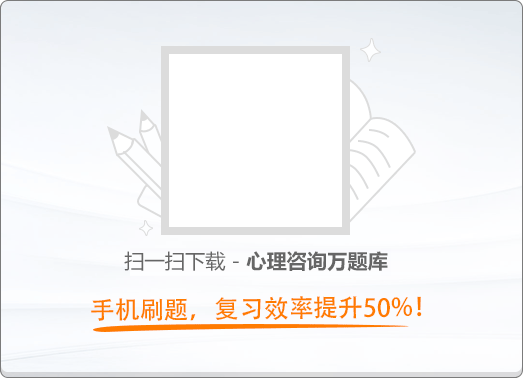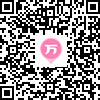90:002015年11月二级心理咨询师《职业道德、理论知识》真题

1
(单项选择题)

• A.

道德是处理人与人、人与社会、人与自然关系的特殊行为规范

• B.

道德是一种理想化的、难以落到实处的行为规范

• C.

道德是一种人人能够认同，但人人又不愿意身体力行的行为规范

• D.

道德是一个缺乏共同评价标准的行为规范

• A
• B
• C
• D

2
(单项选择题)

• A.

谦虚谨慎，戒骄戒躁，脱离低级趣味

• B.

树立正确的权力观、地位观、利益观

• C.

牢记“两个务必”

• D.

“八荣八耻”

• A
• B
• C
• D

3
(单项选择题)

• A.

改革创新观念

• B.

节能减排意识

• C.

团结协作意识

• D.

求真务实精神

• A
• B
• C
• D

4
(单项选择题)

• A.

人是合群的社会性动物，要加强合作和谐，不能搞单打一

• B.

人的心灵需要慰藉，要防止出现曲高和寡的状况

• C.

人在独处时，要谨防发生违背道德要求的意念和行为

• D.

要树立独立人格意识，防止发生侵犯独立人格的现象

• A
• B
• C
• D

5
(单项选择题)

• A.

集体主义

• B.

合理利己主义

• C.

社会主义

• D.

温和功利主义

• A
• B
• C
• D

6
(单项选择题)

• A.

不断强化从业人员追求利益最大化的动机

• B.

严格按照法律矫正员工的动机和行为

• C.

加强制度建设，通过制度建设推动企业行为规范化

• D.

开展职业纪律教育，增强从业人员的纪律意识

• A
• B
• C
• D

7
(单项选择题)

• A.

要不计成本地满足顾客的要求

• B.

无条件地满足顾客提出的任何要求

• C.

一切都是顾客说了算

• D.

顾客的满意程度决定企业的命运

• A
• B
• C
• D

8
(单项选择题)

• A.

只要掌握了高新技术，就能打造品牌

• B.

打造品牌，核心任务是加强广告等媒体宣传工作的力度

• C.

员工具有敬业精神和强烈的社会责任感是打造企业品牌的内在力量

• D.

质优价高才能打造品牌

• A
• B
• C
• D

9
(多项选择题)

• A.

休闲功能

• B.

导向功能

• C.

整合功能

• D.

健身功能

• A
• B
• C
• D

10
(多项选择题)

• A.

职业活动与社会分工密切相关

• B.

职业活动当中的物质生产活动是人类生存的第一个前提

• C.

职业活动包含着高尚的内涵，它绝不能作为人谋生的手段

• D.

职业活动限制了人的自由与发展

• A
• B
• C
• D

11
(多项选择题)

《公民道德建设实施纲要》指出的职业道德规范包括（　）。

• A.

爱岗敬业

• B.

服务群众

• C.

开拓创新

• D.

艰苦奋斗

• A
• B
• C
• D

12
(多项选择题)

• A.

“问别人去”

• B.

“不知道”

• C.

“我有什么办法，又不是我让它坏的”

• D.

“后边等着去”

• A
• B
• C
• D

13
(多项选择题)

• A.

仪表堂堂

• B.

语言规范

• C.

举止优雅

• D.

待人热情

• A
• B
• C
• D

14
(多项选择题)

• A.

慎重选择职业，一旦选择了某种职业就要踏踏实实干一辈子

• B.

强化职业责任，严格履行职业责任的规定

• C.

提高职业技能，不断学习理论知识，提高业务能力

• D.

加强职业责任修养，不断锤炼自己的职业意志

• A
• B
• C
• D

15
(多项选择题)

• A.

甲与乙签订供货合同，后来原料涨价，甲要求提价没有得到同意便中断供货

• B.

张某答应李某“摆平”赵某，后来张某意识到错误，便借故不干了

• C.

X厂发货到Y厂，不知何故Y厂迟迟没有收到货物，X厂依合同进行赔偿

• D.

某女结识某男，后该女发现该男不诚实，于是她采取多种方式愚弄对方

• A
• B
• C
• D

16
(多项选择题)

• A.

工作中相互取长补短

• B.

互助即互相帮助，是否要帮助同事要以对方能否帮助自己为前提

• C.

以自己的兴趣爱好为标准，要求他人向自己看齐.

• D.

做事情多从善良、积极的角度思考问题，树立团队意识

• A
• B
• C
• D